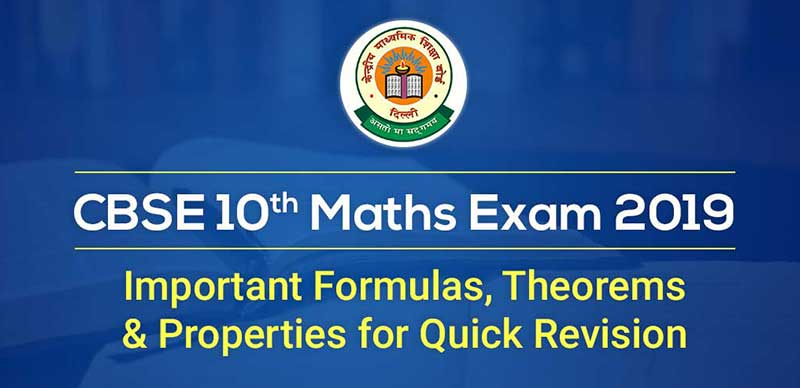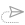### List of Important Formulas, Theorems and Properties for Quick Revision - Class 10th Mathematics

The CBSE Examination for Class 10 Mathematics will be conducted on March 7, 2019. Mathematics is one subject which often gives nightmares to students. While Maths is a little tricky, it is not difficult. It just requires a thorough understanding of concepts, regular practice and a good hold on all important formulas to score high in the Maths subject.

In this article, we have collated all important formulas from all chapters of CBSE Class 10 Maths. We have also put together the important terms and properties which are useful in solving the Class 10 Maths problems.

Important formulas, Terms and properties

1. Real Numbers:

Euclid’s Division Algorithm (lemma): According to Euclid’s Division Lemma if we have two positive integers a and b, then there exist unique integers q and r  such that a = bq + r, where 0 ≤ r ≤ b. (Here, a = dividend, b = divisor, q = quotient and r = remainder.)

2. Polynomials:

(i) (a + b)2 = a2 + 2ab + b2

(ii) (a – b)2  = a2 – 2ab + b2

(iii) a2 – b= (a + b) (a – b)

(iv) (a + b)3  = a3 + b3 + 3ab(a + b)

(v) (a – b)3  =  a3 – b3 – 3ab(a – b)

(vi) a3 + b3 = (a + b) (a– ab + b2)

(vii) a3 – b3  = (a – b) (a+ ab + b2)

(viii) a4 – b4 = (a2)2 – (b2)2 = (a2 + b2) (a2 – b2) = (a2 + b2) (a + b) (a – b)

(ix) (a + b + c) 2  = a2 + b2 + c2 + 2ab + 2bc + 2ac

(x) (a + b – c) 2  =  a2 + b2 + c2 + 2ab – 2bc – 2ca

(xi) (a – b + c)2  = a2 + b2 + c2 – 2ab – 2bc + 2ca

(xii) (a – b – c)2  = a2 + b2 + c2 – 2ab +  2bc – 2ca

(xiii) a3 + b3 + c3 – 3abc  = (a + b + c)(a2 + b2 + c2 – ab – bc – ca)

3. Linear Equations in Two Variables:

For the pair of linear equations

a1 + b1y + c1 = 0  and  a2 + b2y + c2 = 0,

the nature of roots (zeroes) or solutions is determined as follows:

(i) If a1/a2 ≠ b1/b2 then we get a unique solution and the pair of linear equations in two variables are consistent. Here, the graph consists of two intersecting lines.

(i) If a1/a2 ≠ b1/b2 ≠ c1/c2, then there exists no solution and the pair of linear equations in two variables are said to be inconsistent. Here, the graph consists of parallel lines.

(iii) If a1/a2 = b1/b2 = c1/c2, then there exists infinitely many solutions and the pair of lines are coincident and therefore, dependent and consistent. Here, the graph consists of coincident lines.

For a quadratic equation, ax+ bx + c = 0• Sum of roots = –b/a
• Product of roots = c/a
• If roots of a quadratic equation are given, then the quadratic equation can be represented as:

x2 – (sum of the roots)x + product of the roots = 0

• If Discriminant > 0, then the roots the quadratic equation are real and unequal/unique. Here, the graph consists of intersecting lines.
• If Discriminant = 0, then the roots the quadratic equation are real and equal. Here, the graph consists of coincident lines.
• If Discriminant < 0, then the roots the quadratic equation are imaginary (not real). Here, the graph consists of parallel lines.
•

Important Formulas - Boats and Streams

(i) Downstream

In water, the direction along the stream is called downstream.

(ii) Upstream

In water, the direction against the stream is called upstream.

(iii) Let the speed of a boat in still water be u km/hr and the speed of the stream be v km/hr, then

Speed downstream = (u + v) km/hr
Speed upstream = (u - v) km/hr.

5. Arithmetic Progression:

• nth Term of an Arithmetic Progression: For a given AP, where a is the first term, d is the common difference, n is the number of terms, its nth term (an) is given as a= a + (n−1)×d
• Sum of First n Terms of an Arithmetic Progression, Sn is given as:6. Similarity of Triangles:

• If two triangles are similar then ratio of their sides are equal.• Theorem on the area of similar triangles: If two triangles are similar, then the ratio of the area of both triangles is proportional to the square of the ratio of their corresponding sides.7. Coordinate Gemetry:

• Distance Formulae: Consider a line having two point A(x1, y1) and B(x2, y2), then the distance of these points is given as:• Section Formula: If a point p divides a line AB with coordinates A(x1, y1) and B(x2, y2), in ratio m:n, then the coordinates of the point p are given as:• Mid Point Formula: The coordinates of the mid-point of a line AB with coordinates A(x1, y1) and B(x2, y2), are given as:• Area of a Triangle: Consider the triangle formed by the points A(x1, y1) and B(x2, y2) and C(x3, y3) then the area of a triangle is given as-8. Trigonometry:

In a right-angled triangle, the Pythagoras theorem states

(perpendicular )+ ( base )2 = ( hypotenuse )2

Important trigonometric properties are: (with P = perpendicular, B = base and H = hypotenuse)

• SinA = P / H
• CosA = B / H
• TanA = P / B
• CotA = B / P
• CosecA = H / P
• SecA = H/B
• Sin2A + Cos2A = 1
• Tan2A + 1 = Sec2A
• Cot2A + 1 = Cosec2A

Trigonometric Identities:

• sin2A + cos2A=1
• tan2A +1 = sec2A
• cot2A + 1= cosec2A

Relations between trigonometric identities are given below:Trigonometric Ratios of Complementary Angles are given as follows:

• sin (90° – A) = cos A
• cos (90° – A) = sin A
• tan (90° – A) = cot A
• cot (90° – A) = tan A
• sec (90° – A) = cosec A
• cosec (90° – A) = sec A

Values of Trigonometric Ratios of 0° and 90° are tabulated below:9. Circles:

Important properties related to circles:

Equal chord of a circle are equidistant from the centre.

The perpendicular drawn from the centre of a circle, bisects the chord of the circle.

The angle subtended at the centre by an arc = Double the angle at any part of the circumference of the circle.

Angles subtended by the same arc in the same segment are equal.

To a circle, if a tangent is drawn and a chord is drawn from the point of contact, then the angle made between the chord and the tangent is equal to the angle made in the alternate segment.The sum of opposite angles of a cyclic quadrilateral is always 180o.

Important formulas related to circles:• Area of a Segment of a Circle: If AB is a chord which divides the circle into two parts, then the bigger part is known as major segment and smaller one is called minor segment.Here, Area of the segment APB = Area of the sector OAPB – Area of ∆ OAB

10. Mensuration:

Check below the important formulas for areas and volumes of solids:11. Probability:Understanding the basic concepts and learning all the important formulas is extremely sufficient to pass the Maths exam with flying colours. If you know the formulas very well then it will not take much time for you to solve the questions. So, keep practicing with the list of important formulas given above in this article.##### CBSE 10th Term-I Sample Papers 2021-22 : Download Subject-Wise Sample Papers & Marking Scheme For Class-10 Term-I

3 September, 2021, 1:19 pm##### CBSE 10th Case Study Questions 2021-22 : English Chapter-Wise Case Study Questions With Multiple Worksheets & Explanations

3 September, 2021, 11:40 am##### CBSE 10th Case Study Questions 2021-22 : Social Studies Chapter-Wise Case Study Questions With Multiple Worksheets & Explanations

27 August, 2021, 5:36 pm##### CBSE 10th Board Exam 2021-22 : Check Out CBSE 10th Special Study Plan For Term-1 Boards, Point-Wise Details Inside

23 August, 2021, 5:34 pm##### CBSE 10th Case Study Questions 2021-22 : Science Chapter-Wise Case Study Questions With Multiple Worksheets & Explanations

18 August, 2021, 4:20 pm##### CBSE 10th Case Study Questions 2021-22 : Mathematics Chapter-Wise Case Study Questions With Multiple Worksheets & Explanations

14 August, 2021, 3:16 pmWe are providing something unique, useful and most importantly fun. By giving students a tool to find instant solutions to their doubts, we’re trying to make every student self-sufficient in practicing & completing their homework

### COMPANY×